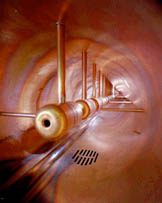# Linear Accelerators

As long ago as 1928, R. Wideroe demonstrated that electrons could be accelerated through a tube by applying a radio frequency voltage to separated sections of the tube so that the electrons felt an accelerating electric field when they passed the gap. If it was arranged so that the electrons arrived at the next gap at the right phase of the RF voltage, they would be accelerated again, getting double the energy they would have gotten from just the application of the maximum field of the RF.

The linear particle accelerator is an extension of Wideroe's idea to a long linear array of accelerating "cells" powered by a radio frequency source in the megawatt power range and in the gigahertz frequency range. Besides adjusting the successive cells so that each one is longer than its predecessor to account for the increasing particle speed, there are subtleties about matching the relative phase of the electromagnetic wave and the particle speed in the accelerator. Present linear accelerators attempt to optimize the acceleration by shaping the waveguide cavity of the accelerator so that the phase velocity of the electromagnetic wave matches the particle speed at the locations where acceleration occurs.

The largest linear accelerator is at the Stanford Linear Accelerator Center (SLAC). It is 3.2 kilometers long and accelerates electrons to 25 GeV. It is also used as an electron-positron collider with total energy 50 GeV per beam.

 A linear accelerator is used as the second stage of the accelerator chain leading to the Tevatron at Fermilab. Negative hydrogen ions are accelerated to 750 keV by a Cockroft-Walton accelerator and then enter the linear accelerator, approximately 150 meters long. Oscillating electric fields accelerate the negative hydrogen ions to 400 million electron volts (400 MeV). Before entering the third stage (the booster), the ions pass through a carbon foil, which removes the electrons, leaving only the positively charged protons.Image courtesy Fermilab
 Types of Accelerators
Index

Particle concepts

Search for elementary particles

Reference
Rohlf
Ch. 16

 HyperPhysics***** Quantum Physics R Nave
Go Back